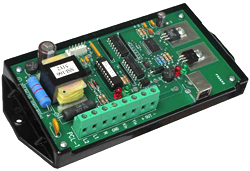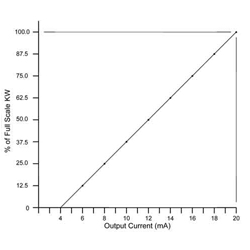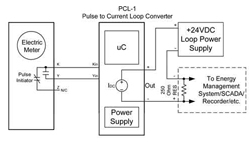# Converting Pulses to an Analog Value

March 2013We’ve been asked hundreds of times for a device that would convert pulses to an analog voltage or current that represented a KW level. Well, finally developed such a device, called the PCL-1. The PCL-1 Pulse-to-Current Loop Converter receives KY pulses from a meter pulse output and converts them to a standard analog 4-20mA output current. The PCL-1 is programmed with the 2-wire pulse constant and a full scale KW value. From there each time a pulse is received, the calculation is done to calculate the instantaneous kW demand. Here’s an example:If a building has a 483KW maximum demand, set the Full Scale Value at 500KW. The absolute maximum is 500KW. Therefore, 500KW = 20mA. 0KW = 4mA. Resolution would be 500 / 255 or 1.961kW (or 3.922% of full scale) per step. Assume that the electric meter’s PKe Pulse value (3 Wire) is 240wh/pulse (.240kWh/pulse) Therefore the 2-wire equivalent pulse value is 480 wh/pulse. Let’s say that pulses right now at this moment in time are coming at a rate of one pulse per 4 seconds and the load is steady. Conversion would be: .480 kWh X 3600 = 1728 kW-sec / 4 sec = 432 kW. Output current would be 432/500 = 86.4% X 16mA = 13.824mA + 4mA = 17.824mA out. Output resolution is 16mA/256 setps or .0625mA per step. So, 256 * 86.4% = 221.184 steps of 256. Rounding off 221 X .0625mA = 13.8125mA + 4mA = 17.8125mA output. Accuracy = 99.9168% The 17.81mA output current would be fed into a device with a 4-20mA current input which contains a precision 250 ohm .1% resistor which converts the current into a usable voltage between 1.0VDC and 5.0VDC which is readable by the devices’ A-D converter.In addition to converting the pulse to an instantaneous kW level, the PCL-1 contains an Average output mode that can output a current that represents the calculated kW average similar to the electric meter’s Average kW calculation on a 1, 2 5, 10, 15, 30, or 60 minute average interval. The PCL-1 is programmed using a free software program called the pcl-host.exe and communicates using the PCL-1’s USB port. In summary, the PCL-1 is a simple and easy, low cost way to convert pulses to a usable analog voltage level that can be used by many systems. Learn more about the PCL-1 Pulse-to-Current Loop Converter
Previous Article: On the Road Again